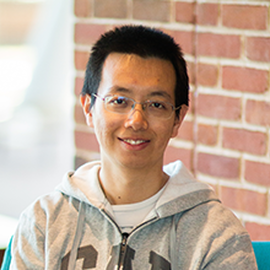# Digital quantum simulation by product formulas (TQC 2019)### Abstract

Merged talk.

Faster quantum simulation by randomization:
Product formulas can be used to simulate Hamiltonian dynamics on a quantum computer by approximating the exponential of a sum of operators by a product of exponentials of the individual summands. This approach is both straightforward and surprisingly efficient. We show that by simply randomizing how the summands are ordered, one can prove stronger bounds on the quality of approximation for product formulas of any given order, and thereby give more efficient simulations. Indeed, we show that these bounds can be asymptotically better than previous bounds that exploit commutation between the summands, despite using much less information about the structure of the Hamiltonian. Numerical evidence suggests that the randomized approach has better empirical performance as well.

Nearly optimal lattice simulation by product formulas:
We consider simulating an $n$-qubit Hamiltonian with nearest-neighbor interactions evolving for time $t$ on a quantum computer. We show that this simulation has gate complexity $(nt)^{1+o(1)}$ using product formulas, a straightforward approach that has been demonstrated by several experimental groups. While it is reasonable to expect this complexity—in particular, this was claimed without rigorous justification by Jordan, Lee, and Preskill—we are not aware of a straightforward proof. Our approach is based on an analysis of the local error structure of product formulas, as introduced by Descombes and Thalhammer and further simplified here. We prove error bounds for canonical product formulas, which include well-known constructions such as the Lie-Trotter-Suzuki formulas. We also develop a local error representation for time-dependent Hamiltonian simulation, and we discuss generalizations to periodic boundary conditions, constant-range interactions, and higher dimensions. Combined with a previous lower bound, our result implies that product formulas can simulate lattice Hamiltonians with nearly optimal gate complexity.

Date
Jun 5, 2019
Location
University of Maryland, College Park, MD 20742, United States##### Yuan Su
###### Senior Researcher

I work on quantum algorithms for simulating Hamiltonian dynamics. I am particularly interested in the design, analysis, implementation, and application of quantum simulation.